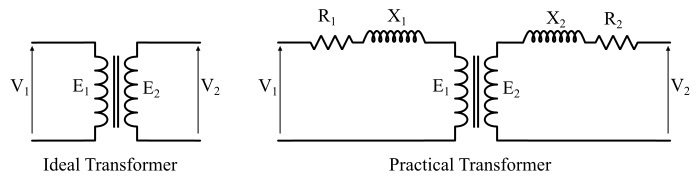# Difference between Ideal Transformer and Practical Transformer

A transformer is a static electrical machine which is used to transform the level of alternating voltage, i.e. a transformer increases or decreases the value of alternating voltage without changing in its frequency.

As every electrical machine has some power loss in it, thus depending upon the losses occur in the transformer, the transformer is of two types viz. −

• Ideal transformer

• Practical transformerIn this article, we will enlist all the significant differences between ideal transformer and practical transformer. We have also added a short description of ideal transformer and practical transformer for your reference.

## What is an Ideal Transformer?

The theoretical model of a real transformer without losses is known as ideal transformer. In other words, an ideal transformer is an imaginary transformer for which the energy losses are zero.

An ideal transformer has the following characteristics −

• The primary and secondary windings have negligible (or zero) resistance.

• No leakage flux, i.e., whole of the flux is confined to the magnetic circuit.

• The magnetic core has infinite permeability, thus negligible MMF is require to establish flux in the core.

• There are no losses due winding resistances, hysteresis and eddy currents. Hence, the efficiency is 100%.

It is important to note that an ideal transformer does not exist in real life, which means it is a hypothetical and cannot be realized practically.

## What is a Practical Transformer?

A practical transformer is one in which there are some energy losses inside the transformer due to its core and winding characteristics. In simple words, a transformer which has some loss of energy in it is known as practical transformer or real transformer. Therefore, the practical transformer can be represented by the following equation,

Ideal Transformer + Energy Losses = Practical Transformer

The main characteristics of a practical transformer are as follows −

• The primary and secondary windings have finite resistance.

• There is a leakage flux, i.e., whole of the flux is not confined to the magnetic circuit.

• The magnetic core has finite permeability, thus a considerable amount of MMF is require to establish flux in the core.

• There are losses in the transformer due to winding resistances, hysteresis and eddy currents. Therefore, the efficiency of a practical transformer is less than 100 %.

All the transformers that we achieve in real world are practical transformers, which have some energy losses and the efficiency less than 100%.

## Difference between Ideal Transformer and Practical Transformer

The key differences between ideal transformer and practical (or real) transformer are highlighted in the following table −

Basis of Difference
Ideal Transformer
Practical Transformer
Definition
An ideal transformer is a theoretical model of transformer with no energy losses in it.
A practical transformer is one which has energy losses in it.
Core losses
For an ideal transformer, the core losses (i.e. hysteresis loss and eddy current loss) are zero.
A practical transformer has finite core losses.
Copper losses
The copper loss (or I2R loss) in an ideal transformer is zero.
There is finite copper loss in case of a practical transformer.
Efficiency
The efficiency of an ideal transformer is equal to 100%.
A practical transformer always has efficiency less than 100%.
Dependency of efficiency
The efficiency of an ideal transformer is always 100% and does not depend on any parameter.
The efficiency of a practical transformer depends upon the power factor and loading of the transformer.
Winding resistance
The resistance of the windings of an ideal transformer is zero (or negligibly small).
The windings of a practical transformer have some finite resistance.
Ohmic resistance drop
In an ideal transformer, there is no Ohmic resistance drop due to zero winding resistance.
A practical transformer has some Ohmic resistance drop due to winding resistance
Nature of winding coils
The windings of an ideal transformer are purely inductive.
The windings of a practical transformer are not purely inductive, they also have a finite resistance.
Magnetic flux leakage
There is no leakage flux in an ideal transformer, which means all the flux produced by primary winding gets fully linked to the secondary winding.
There is a flux leakage in a practical transformer.
Permeability of core
The permeability of core of an ideal transformer is considered infinite.
The core of a practical transformer has finite permeability.
Existence
An ideal transformer is theoretical model of the transformer, hence it does not exist in real life.
All the transformers exist in real world are the practical transformers.
Applications
An ideal transformer is only used for analyzing the transformer circuits.
The practical transformer is used in practice to step-up or step-down the voltage levels.

## Conclusion

In this article, we explained all the noticeable differences between ideal transformer and practical transformer by considering various parameters such as basic definition, energy losses, existence, efficiency, core permeability, applications, etc. The most significant difference between the ideal transformer and practical transformers is that an ideal transformer is 100% efficient, while the practical transformer can never have 100% efficiency.